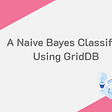# GANs on the Azure (ML) Sea — Part 1

Wouldn’t it be cool if you could have your work done for you by a machine? Put some parameters in, then walk away, come back, and voila — fully formed homework!

For the low sum of just \$19.99\month, you can! If you click the link below, you can sign up for the monthly work-a-holic plan! All you need to do is manually tune your data, your net, and find a way to run things, and boom. Everything else will be taken care of automatically.

In every neural net, there are 3 things that are necessary to properly train an inference engine:

1. Training Data — painstakingly made with the more data the better
2. A neural network — hand crafted with love and care by a data scientist. (That’s what I want to call myself these days!)
3. A way to find the best parameters for your neural network.

Or, at least, that’s all they need at a high level.

Neural networks require massive amounts of training data, the more the better. While there is an ongoing amount of research in allowing neural nets to train on low amounts of data, for now, let’s take the opposite track.

What if we could have a neural network generate the data to train another neural network?

Exactly.

So how do we do this?

Well, first we need to understand what a GAN is:

• G — Generative
• N — Network

Essentially, a GAN is a network that trains itself by fighting itself!

Calling it a network is a poor choice of words. It’s actually a *system* that trains itself.

First, you have the generator neural network — this is trained to try and generate whatever it is you’re looking for. If you’re looking for art, you train it on art.

Second, you have a discriminator. This network is trained to tell what is or isn’t something. So, if you want art, you train it on art. When it can tell you what art is or isn’t, you’re done. (If you can manage to train something to identify art, please call me. Quickly.)

Lastly, you pit them against each other.

The generator sends stuff to the discriminator. The discriminator judges the output and tells the generator what it thinks. And around and around they go, until the generator can fool the discriminator, and (theoretically) the discriminator is just guessing and has a 50\50 shot of being right.

At that point, you’re done!

First, let’s define a new script, ‘train-dcgan.py’. In this script, we’ll do our normal routine, getting the script working locally, and then pushing it over to Azure. Along the way, we’ll push our data to the cloud, manage our costs, and take over the world! (Note — this article is too long, so a part 2 will cover going to Azure.)

This DCGAN is based on the PyTorch tutorial — the intent is to get this running simply on Azure ML https://pytorch.org/tutorials/beginner/dcgan_faces_tutorial.html

It will use a Convolution Neural Network (CNN). If you’re not familiar with CNNs, check out this link: https://ujjwalkarn.me/2016/08/11/intuitive-explanation-convnets/ Essentially, CNNs are generally employed to do image recognition. They take an image in, and apply a mathematical operation called convolution. This creates a smaller and smaller image until only a few data points are left, after which the classification engine kicks in.

In DCGAN, we’ll flip that on its head, and start with a small input, then build up a large output which represents our final image.

Before we get started, we should define a set of parameters for our setup:

• workers — This is the number of worker threads that load data into the DataLoader. In general, we want to set this to the number of CPU threads for a rough estimate. This can be affected by the number of other things you are running on your system. However, since this will effectively run on a dedicated machine in Azure, let’s set this to be dynamic.
• batch size — this is the batch size we are going to use. A batch size defines how many images are used at a time in a training run. By splitting a training set up into multiple via the batch size, we can avoid updating our parameters too rapidly. Effectively, we run through a full batch, then we update internal parameters. This differs from epoch in that an epoch is a complete pass through the training data, updating the parameters at every batch size completion.
• image size — this is the size of the images that the net will use. Images will get resized to this size using the PyTorch resize capabilities. While it sounds simple to use larger images (bigger is better, right?) this actually doesn’t work out this way. The image size is directly tied to the number of features and the number of layers in the neural net. Changing this may cause the network to become unstable and or not arrive at the same results.
• z latent vector — this is effectively the input of the Convolution Neural Net(CNN). It tells the input how large (how many data points) to expect here. Since the output of this generator is an image, and not a classifier, we’re just going to pass some random values to it and have it give us something back — eventually the CNN will make an image with the noise we give it. A more traditional CNN would take an image as its input and provide outputs that classify the image.
• We define 2 variables for the feature maps. Feature maps are essentially the output of the convolution exercise. You can think of them as the data that is stored from one pass through the convolution layer to the next. In our case though — we’re using them to store the actual image itself that we are slowly creating. Over time, we’ll store less and less features (every pass), until we have just the final 64 features, creating our 64 x 64 image.
• Training epochs — how many passes through the data will we take?
• Optimizer learning rate — how much we’ll update the optimizers. These are pretty much set by the DCGAN paper, so go nuts and experiment with this.
• Beta1 hyperparameter— The adam optimizer has 4 hyperparameters — alpha, beta1, beta2, and epsilon. This control how fast the function learns, decays, and prevents division by zero. Note that these values are different than the defaults in PyTorch.
• ngpu — this effectively determines if the code will use the GPU or the CPU. We’ll want to set this dynamically, since we have different Compute Targets, including local, which can be different configurations.

Let’s go ahead and run this:

Okay — everything looks good — but we are missing our GPU.

No worries!

WSL2 drivers from Nvidia are available to developer folks to fix this.

Just follow the directions there, and you’re all set.

Now, let’s move on to the data set — so we can set that up.

We’ll be using the Celeb Faces A set, available at: https://www.kaggle.com/jessicali9530/celeba-dataset

Because we are working with WSL, we’ll need to put this into the WSL set.

If you’re comfortable with Linux, just do the following:

`cd datamkdir celebacd celebaunzip /mnt/c/Documents\ and\ Settings/allan/Downloads/archive.zip`

Note — in the above path, replace ‘allan’ with your Windows user id.

If you’re not that comfortable, then use Windows explorer, open the zip file, and copy it using the Z: mount point we made earlier.

(If your mount point is gone, then do this:

`net use z: \\wsl\$\Ubuntu-20.04`

Then you can use explorer to get to the data directory under your Repository base directory.

It’s important to keep it under your data dir — we’ll want to prevent Azure from uploading the data using the ignore file. We’re going to upload it ourselves in a bit.

When you are done, you should have a directory structure that looks like this:

data \ celeba \ img_align_celeba

We’ll pass ‘data \ celeba ‘ to the dataroot in our training script, and PyTorch will do the heavy lifting of loading it up.

Let’s load that data into our script!

`# Create the datasetdataset = dset.ImageFolder(root=dataroot,transform=transforms.Compose([transforms.Resize(image_size),transforms.CenterCrop(image_size),transforms.ToTensor(),transforms.Normalize((0.5, 0.5, 0.5), (0.5, 0.5, 0.5)),]))`

First — ImageFolder is one of the supported data sets for PyTorch. What this does is set up a folder of images with a generic loader.

In loading them in, you can specify a transform — this transform will ensure that all images are the same.

In machine learning Data is extremely important. One particular example of bad data was in an Army Research project — they were building a neural net to detect tanks. It had a 100% accuracy rate… right up till the live demo. It failed every miserably.

It turns out, that since every picture of a tank they trained the neural net with was taken on a rainy day… they had built an expensive rain detector.

In this case, we are going to resize every image down to the same size — which matches our image size variable we set earlier.

We are then going to crop them, and turn them into a PyTorch Tensor. Sounds pretty tense!

A PyTorch tensor is effectively a a numpy array wrapper — although, through the magic of objects, each tensor can be run on either the GPU or CPU. That is, these are special arrays with new overloaded methods, which can take advantage of the underlying machine for their accelerations.

The last step here prepares the data for consumption by the neural net — normalizing it between -1 and 1. This is done so that when we multiply our gradients by the learning rate, we don’t get some values swinging wildly out of range. Ie — if a value was 5, when multiplied by 2, it would end up 10, but a value of 1 would be 2. The 10 has 5x the pull the the 2 does now. It keeps things a bit more even throughout the whole learning process.

You’ll notice that when we add this last set of code, using the device, that pyLint throws an error. This error is related to: https://github.com/pytorch/pytorch/issues/701 and comes because pyLint can’t match the function we are using to the function export in the library.

To fix this, add the following to your User Settings in VS Code:

You can get there by File->Preferences->Settings, then clicking Extensions->Python.

`device = torch.device("cuda:0" if (torch.cuda.is_available() and ngpu > 0) else "cpu")`

This line sets up the device to be either cpu or gpu. As of now, only Nvidia GPUs are supported.

It is also the line that will start throwing pyLint errors.

Lastly — this line:

`plt.imshow(np.transpose(vutils.make_grid(real_batch.to(device)[:64], padding=2, normalize=True).cpu(),(1,2,0)))`

This line in a normal scenario would show a GUI popup with an image. However, in WSL, we’d have to install a GUI environment. So, instead, we’ll go ahead and save an image instead.

`images = vutils.make_grid(real_batch.to(device)[:64], padding=2, normalize=True)vutils.save_image(images, 'sample.png', normalize=True)`

That’s it! It will save an image in the src dir — sample.png. We’ll go ahead and comment this out in a bit, but you can check we’re loading things in. :)

The ‘real_batch’ syntax is because our real_batch has a length of ‘data points \ batch size’. We’re just testing the waters here, so let’s just show we loaded some data. :)

And Voila — our data is loaded and initialized.

Next we need to setup our Generator. The Generator is what will try and make a new face.

The generator is composed of 5 layers, the magic here is:

`nn.BatchNorm2d(ngf * 2),`

this call, between every Convolution Transpose and ReLU operation, normalizes the input again. According to the smart people that wrote this paper, it’s the “magic”. :)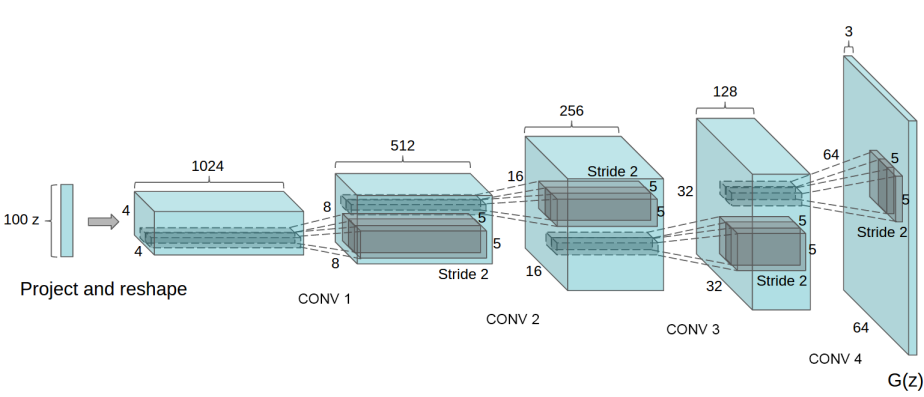The 5 layers of the generator, eventually resulting in the final 64 x 64 image.

The rest of the code can be found in the class Generator in train-dcgan.py.

Let’s go ahead and instantiate the Generator:

`# Create the generatornetG = Generator(ngpu).to(device)# Handle multi-gpu if desiredif (device.type == 'cuda') and (ngpu > 1):    netG = nn.DataParallel(netG, list(range(ngpu)))# Apply the weights_init function to randomly initialize all weights#  to mean=0, stdev=0.2.netG.apply(weights_init)# Print the modelprint(netG)`

This code will go ahead and instantiate the Generator, as well as run initialize our initial weights. We’ll print out the model to ensure that everything is working.

`Generator(  (main): Sequential(    (0): ConvTranspose2d(100, 512, kernel_size=(4, 4), stride=(1, 1), bias=False)    (1): BatchNorm2d(512, eps=1e-05, momentum=0.1, affine=True, track_running_stats=True)    (2): ReLU(inplace=True)    (3): ConvTranspose2d(512, 256, kernel_size=(4, 4), stride=(2, 2), padding=(1, 1), bias=False)    (4): BatchNorm2d(256, eps=1e-05, momentum=0.1, affine=True, track_running_stats=True)    (5): ReLU(inplace=True)    (6): ConvTranspose2d(256, 128, kernel_size=(4, 4), stride=(2, 2), padding=(1, 1), bias=False)    (7): BatchNorm2d(128, eps=1e-05, momentum=0.1, affine=True, track_running_stats=True)    (8): ReLU(inplace=True)    (9): ConvTranspose2d(128, 64, kernel_size=(4, 4), stride=(2, 2), padding=(1, 1), bias=False)    (10): BatchNorm2d(64, eps=1e-05, momentum=0.1, affine=True, track_running_stats=True)    (11): ReLU(inplace=True)    (12): ConvTranspose2d(64, 3, kernel_size=(4, 4), stride=(2, 2), padding=(1, 1), bias=False)    (13): Tanh()  ))`

Our next step is the Discriminator. As mentioned earlier, the Discriminator is the thing that tells the Generator whether it made something good… or something totally fake. Again, the full Discriminator class can be found in the train-dcgan.py file.

`netD = Discriminator(ngpu).to(device)# Handle multi-gpu if desiredif (device.type == 'cuda') and (ngpu > 1):    netD = nn.DataParallel(netD, list(range(ngpu)))# Apply the weights_init function to randomly initialize all weights#  to mean=0, stdev=0.2.netD.apply(weights_init)# Print the modelprint(netD)Discriminator(  (main): Sequential(    (0): Conv2d(3, 64, kernel_size=(4, 4), stride=(2, 2), padding=(1, 1), bias=False)    (1): LeakyReLU(negative_slope=0.2, inplace=True)    (2): Conv2d(64, 128, kernel_size=(4, 4), stride=(2, 2), padding=(1, 1), bias=False)    (3): BatchNorm2d(128, eps=1e-05, momentum=0.1, affine=True, track_running_stats=True)    (4): LeakyReLU(negative_slope=0.2, inplace=True)    (5): Conv2d(128, 256, kernel_size=(4, 4), stride=(2, 2), padding=(1, 1), bias=False)    (6): BatchNorm2d(256, eps=1e-05, momentum=0.1, affine=True, track_running_stats=True)    (7): LeakyReLU(negative_slope=0.2, inplace=True)    (8): Conv2d(256, 512, kernel_size=(4, 4), stride=(2, 2), padding=(1, 1), bias=False)    (9): BatchNorm2d(512, eps=1e-05, momentum=0.1, affine=True, track_running_stats=True)    (10): LeakyReLU(negative_slope=0.2, inplace=True)    (11): Conv2d(512, 1, kernel_size=(4, 4), stride=(1, 1), bias=False)    (12): Sigmoid()  ))`

The Discriminator is very similar to the Generator, except it uses a LeakyReLU instead of a ReLU.

ReLU vs Leaky ReLU

ReLU stands for rectified linear unit. it’s essentially y = max(0, x). Easy!

• ReLU — Linear — easy to calculate, no complicate math.
• Leaky ReLU — In ReLU, if a neuron gets negative, it probably won’t recover — and thus, it is “dead”. Leaky ReLU allows for neurons to go negative, but uses a small amount of change for negative values, thus better allowing them to recover. The equation becomes y=ax when < 0, where a = .01 usually.

For this tutorial, we’re going to use Loss as our parameter for training. As we’ve seen earlier — loss is the amount of errors made for each training run.

We’re going to pick the Binary Cross Entropy product as our loss function, defined in PyTorch as:

This function, broken down extremely well at: https://towardsdatascience.com/understanding-binary-cross-entropy-log-loss-a-visual-explanation-a3ac6025181a essentially says that we sum the probability that something is labeled correctly, with the probability that it is labeled incorrectly. We do this for all data points in the set.

`# Initialize BCELoss functioncriterion = nn.BCELoss()# Create batch of latent vectors that we will use to visualize#  the progression of the generatorfixed_noise = torch.randn(64, nz, 1, 1, device=device)# Establish convention for real and fake labels during trainingreal_label = 1.fake_label = 0.# Setup Adam optimizers for both G and DoptimizerD = optim.Adam(netD.parameters(), lr=lr, betas=(beta1, 0.999))optimizerG = optim.Adam(netG.parameters(), lr=lr, betas=(beta1, 0.999))`

This code sets up the BCELoss, and generates random (Gaussian) noise to be passed to each generator. Our labels in the BCE function are 0 and 1 — real and fake.

Now that the entire GAN is setup — it’s time to start training it!

Training a GAN is actually not easy. Because of that, I’m not going to deviate much from the PyTorch tutorial or the paper. Obviously, there are a set of best practices, listed here: https://github.com/soumith/ganhacks

The first thing we need to do is to setup the Discriminator. This will allow us to have something to train the Generator against later. The Discriminator will be trained against minibatches — each batch will consist of either real or fake images. The fake images will be generated by the Generator.

The Generator will be trained by computing a Loss of the Real images. This allows us to compare the Generator’s output against real images. Eventually, the Generator will be fed into the Discriminator and they will train each other!

Lastly, of course, we’ll start the process of measuring things. This will become especially important on Azure — we’ll want to track metrics like this.

So, for now, we’ll just print out some training statistics when we run:

• Loss_D — the sum of the losses for the real and fake batches for the Discriminator
• Loss_G — The Generator Loss
• D(x) — the output of the Discriminator for the all real batch. This essentially tells us whether the input was real or fake. This will start at 1 (fake), and move towards (.5) as the Generator gets better. .5 is essentially the Discriminator flipping a coin, and represents our best output for the fake batch.
• D(G(x)) — this is the Discriminator output for the the all fake batch. It will start at 0 — everything is fake! — and move to .5 — I dunno… 50\50 shot at guessing? As the Generator gets better, it will affect the Discriminator’s performance on the real batch, until both batches are essentially 50\50.

The code is too long to paste here — so take a look at ‘train-dcgan.py’.

Go ahead and run it. On my system, it takes about 116m.

And, here’s the output! You can see the pictures start as Gaussion noise (from our input z!) and get closer and closer to something that looks like faces.

Okay — it’s not great. Honestly, more training, etc could probably be used. But, our point here is not just to train the heck of out of this thing — but to move it to Azure.

Which, because I’ve just hit the limit for an Article, will have to wait till Part 2!

--

--

--

## More from Allan Graves

Years of technology experience have given me a unique perspective on many things, including parenting, climate change, etc. Or maybe I’m just opinionated.

Love podcasts or audiobooks? Learn on the go with our new app.

## Why Deep Learning is Now Easy for Data Scientists |## A Neural Network that Can Tell the Genres of a Movie## Implementing Chargrid: Towards Understanding 2D Documents.## Human-Centric & Interpretable Machine Learning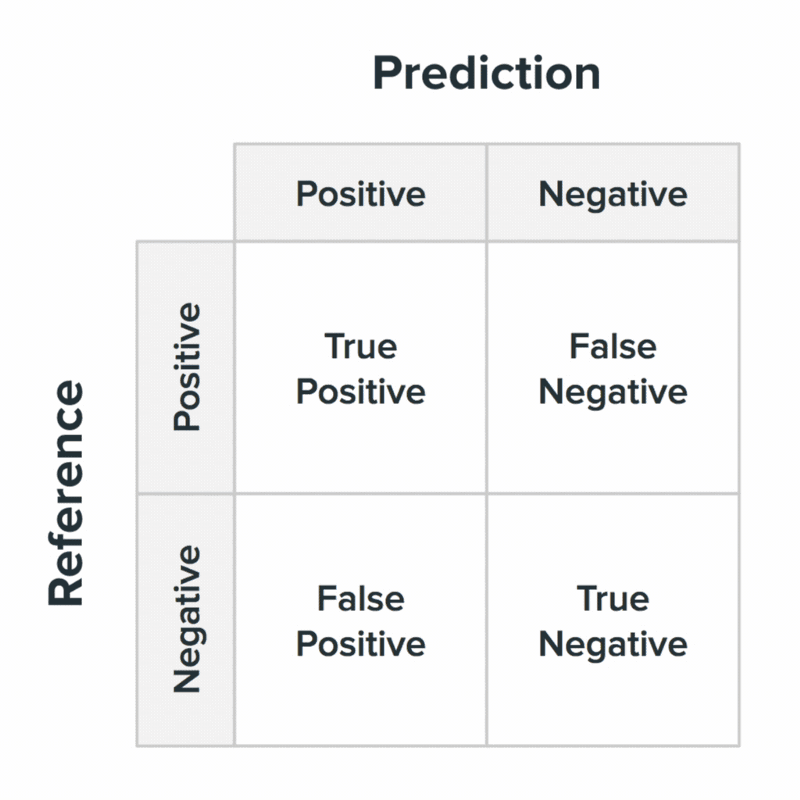## Faster Drilling with Machine Learning and Particle Swarm Optimization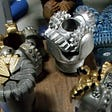## Trading Through Reinforcement Learning using LSTM Neural Networks## How we used AI to optimize software for supercomputers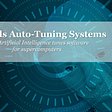## Meet Matterport’s newest employee: Thomas Bayes## Allan Graves

Years of technology experience have given me a unique perspective on many things, including parenting, climate change, etc. Or maybe I’m just opinionated.

## Python script to push sample data to InfluxDB## Hello viewers, I am PANEENDRA V.S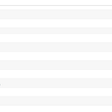## LightGBM Starter Code## A Naive Bayes Classifier Using Java & GridDB | GridDB: Open Source Time Series Database for IoT